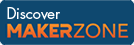Cody

# Problem 1. Times 2 - START HERE

Created by Cody Team in Community

Try out this test problem first.

Given the variable x as your input, multiply it by two and put the result in y.

Examples:

``` Input  x = 2
Output y is 4```
``` Input  x = 17
Output y is 34```

Press the "Solve This Now!" button below to get started...

### Solution Stats

72.56% Correct | 27.44% Incorrect
Last solution submitted on Mar 19, 2019

#### TagsMATLAB and Simulink resources for Arduino, LEGO, and Raspberry Pi